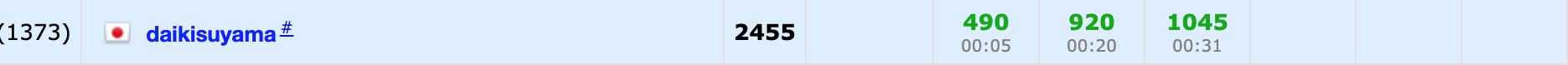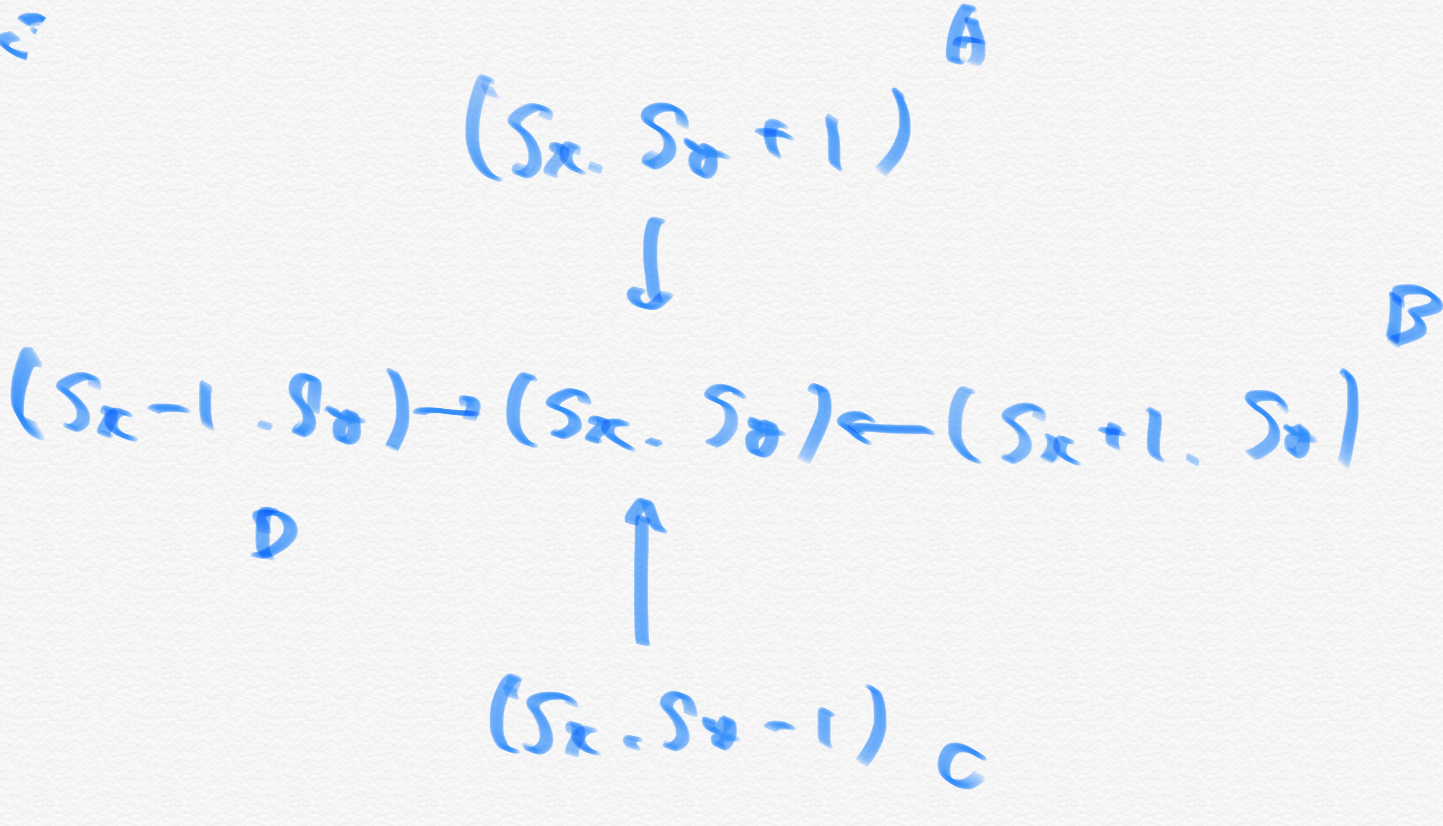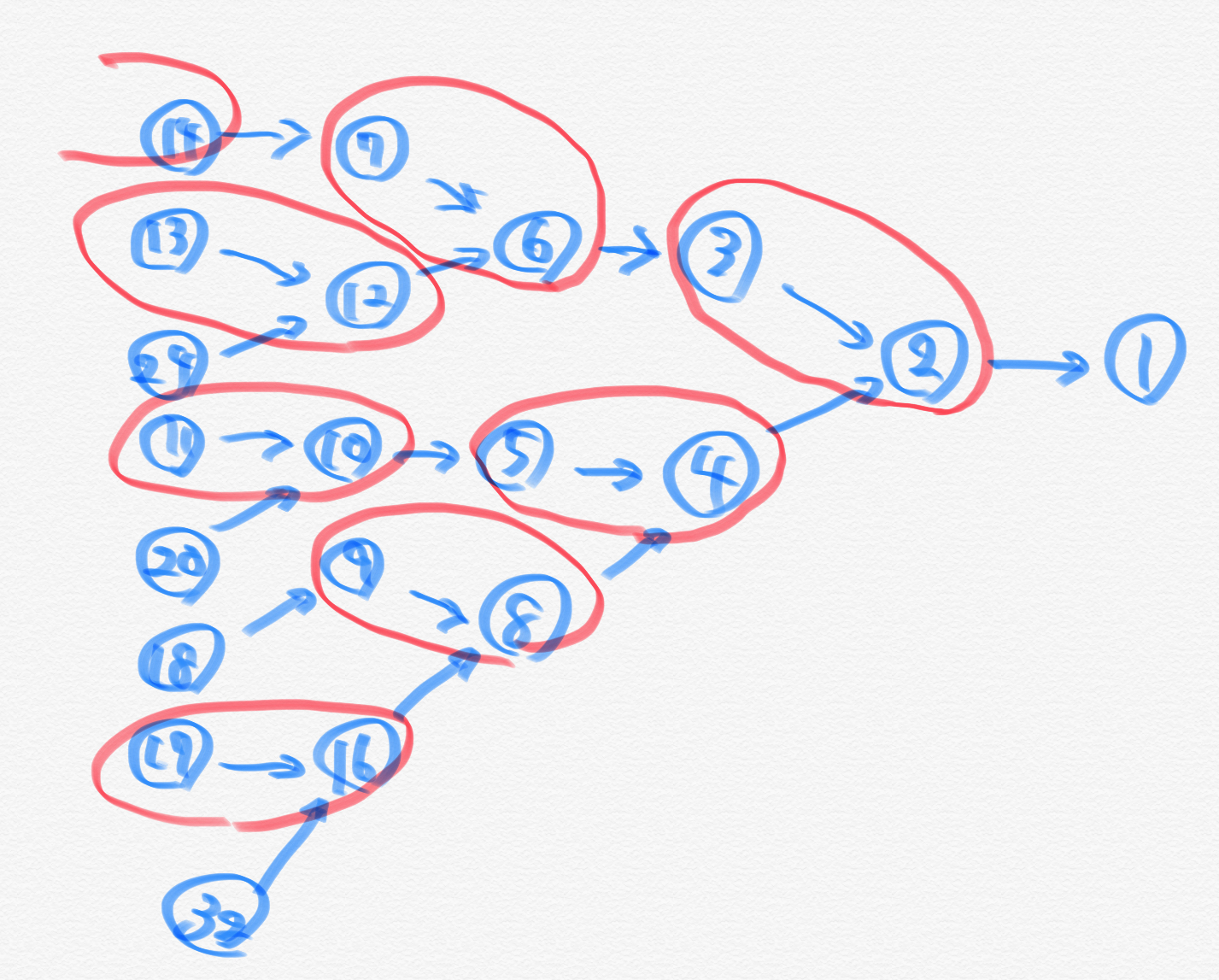# This time's results# Impressions of this time

It was early until the C problem, but from there ... I have to say that I should do my best because the D problem was too long and I was discouraged from reading it, but it is quite a reflection that I could not pass the E problem after an hour and a half. I have written down the points of reflection in the E problem, so I will be careful after that.

# Problem A

Since \$ d \$ is covered, consider which is the best to allocate **. At this time, if it is \$ e > f \$, it is best to sort it into the first type, and if it is \$ e \ <f \$, it is best to sort it into the second type. When \$ e = f \$, it doesn't matter which one you use.

Therefore, if you consider the cases by \$ e > f \$, \$ e \ <f \$, it will be as follows.

(1) In the case of \$ e > f \$ It is best to choose the first type, only \$ m = min (a, d) \$. Also, since the rest of \$ d \$ is \$ d-m \$, you can select only \$ min (b, c, d-m) \$ for the second type.

(2) In case of \$ e \ <f \$ It is best to choose the first type, only \$ m = min (b, c, d) \$. Also, since the rest of \$ d \$ is \$ d-m \$, you can select only \$ min (a, d-m) \$ for the second type.

#### `A.py`

``````
a,b,c,d,e,f=[int(input()) for i in range(6)]
if e<f:
m=min(b,c,d)
ans=m*f
b-=m
c-=m
d-=m
ans+=min(a,d)*e
else:
m=min(a,d)
ans=m*e
a-=m
d-=m
ans+=min(b,c,d)*f
print(ans)

``````

# Problem B

(Hereafter, black is B and white is W.)

You can do it up to \$ 3n \$, so consider building it conveniently. Also, we will operate so that all colors are the same, but we will consider unifying to ** B . Also, if you perform the operation to invert the \$ i, i + 1 \$ th only when the \$ i \$ th is W in order from the smallest \$ i \$, ** Always keep the B before the \$ i \$ th You can do. At the end of this operation, there are two ways: \$ BB… BB, BB… BBW \$. In the former case, the colors are already unified, so the operations up to this point are output. In the latter case, when B is an even number, you can make the whole W by selecting B appropriately. Also, in the latter case, if B is an odd number, it is impossible (because both \$ \ because \$ B and W have an even number of changes).

Also, the above is at most \$ 2n \$ times, so the condition is met.

#### `B.py`

``````
n=int(input())
s=list(input())
ans=[]
if s=="W":
ans.append(0)
s="B"
if s=="W":
s="B"
else:
s="W"
for i in range(1,n-1):
if s[i]=="W":
s[i]="B"
if s[i+1]=="W":
s[i+1]="B"
else:
s[i+1]="W"
ans.append(i)
if s!=["B" for i in range(n)] and n%2==0:
print(-1)
exit()
if s!=["B" for i in range(n)]:
for i in range(n-1):
if i%2==0:
ans.append(i)

print(len(ans))
print(" ".join(map(str,[i+1 for i in ans])))
``````

# C problemYou can reach \$ (s \ _x, s \ _y) \$ via any of the above four points, and ** when you pass one point, the other points do not pass **, so these four points You can put a tent in one of them.

Considering that you can reach it in the shortest distance,

(1) Coordinates of the house passing through A → \$ y \$ coordinates are \$ s \ _y + 1 \$ or more (2) Coordinates of the house passing through B → \$ x \$ coordinates are \$ s \ _x + 1 \$ or more (3) Coordinates of the house passing through C → \$ y \$ coordinates are \$ s \ _y-1 \$ or less (4) Coordinates of the house passing through D → \$ x \$ coordinates are less than \$ s \ _x-1 \$

Since it is sufficient to satisfy, find the maximum value when each is counted.

#### `C.py`

``````
import sys
n,sx,sy=map(int,input().split())
a,b,c,d=0,0,0,0
for i in range(n):
x,y=map(int,input().split())
if x<=sx-1:
a+=1
elif x>=sx+1:
b+=1
if y<=sy-1:
c+=1
elif y>=sy+1:
d+=1
m=max(a,b,c,d)
print(m)
if a==m:
print(sx-1,sy)
elif b==m:
print(sx+1,sy)
elif c==m:
print(sx,sy-1)
else:
print(sx,sy+1)
``````

# D problem

The problem was hard to read. I will skip this time.

# E problem

There are three main issues to consider in this issue:

(1) ** Experiment is appropriate ** → Instead of "I want to find ** 〇〇 properties **, I will do △△", but "I don't know, so I will do △△ for the time being". (2) ** It is appropriate to cut off the policy ** → ** I can't tell if it's different ** logically ** (3) ** The memo is dirty ** → ** The direction of consideration will be different **

I will write a commentary while checking the above.

It's difficult to count if each number of paths contains \$ z \$, so ** consider what the number of paths contains \$ z \$ **.

For example, ** experiment ** with \$ z = 1 \$ and: … (3)You can see that the numbers appear in a radial pattern, but you can't grasp the law even if you consider only the case of ** \$ z = 1 \$ **, so consider the case of \$ z = 4 \$ and \$ z = 4 Consider the numbers that have paths containing \$ in ascending order. … (1) Then you can see that numbers such as 4 ~ 5 → 8 ~ 11 → 16 ~ 23 →… include \$ z \$ in the path. Generalizing this, we can see that the numbers contained in \$ [z, z + 1], [2z, 2z + 3], [4z, 4z + 7]… \$ have paths containing \$ z \$ ( ✳︎). Also, if \$ z \$ is even, it will be as above, but if it is odd, double it to make it even and then count **.

Therefore, even if you count how many \$ z \$ are included in the path up to \$ n \$, the number of intervals is about a constant multiple of \$ \ log {n} \$, so the number including \$ z \$ in the path is \$ O. You can count with (\ log {n}) \$. In addition, \$ [z, z + 1], [2z, 2z + 3], [4z, 4z + 7]… \$ is smaller than \$ z \$ because more numbers contain \$ z \$ in the path * * Has monotony **. Also, since the counting method is different for even numbers and odd numbers, it is necessary to perform a binary search for each even number and odd number **.

From the above, if the function of counting the number including \$ z \$ in the path is \$ calc (z) \$ (returning is a bool value of \$ k \$ or more even if counted), \$ calc (z) \$ is Since it is True when \$ z \$ is small and False when it is large, the maximum \$ z \$ that is True is calculated by binary search. It is a little troublesome to divide the case by chance, but it is not difficult to implement if you refer to this article. ** You only need to be careful about the definitions of the boundary values \$ l, r \$ **. Also, note that the previous article is looking for the minimum value and this time it is looking for the maximum value, so it is necessary to replace all the roles of \$ l and r \$.

(✳︎)… I will omit the proof, but when \$ z \$ is an even number, it is clear that the number of intervals \$ [z, z + 1] \$ can be expressed, so find the number recursively from here. You can see it.

#### `E.py`

``````
n,k=map(int,input().split())
def calc(z):
global n
if z>n:
return False
if z==0:
return True
if z%2==1:
ans=1
now=2*z
co=1
if k==1:
return True
elif now>n:
return False
else:
ans=0
now=z
co=1
#print(ans,now,co)
while True:
#print(n,now+2*co-1,now)
#print(n-(now)+1)
if n<=now+2*co-1:
ans+=max(0,n-(now)+1)
#print(ans)
break
else:
now*=2
co*=2
ans+=(co)
#print(ans)
#print(ans)
return ans>=k
realans=[]
#odd(2x-1)
l=1
r=n//2+2
while l+1<r:
y=l+(r-l)//2
if calc(2*y-1):
l=y
else:
r=y
realans.append(2*l-1)
#even(2x)
l=0
r=n//2+2
while l+1<r:
y=l+(r-l)//2
if calc(2*y):
l=y
else:
r=y
realans.append(2*l)
print(max(realans))
``````

# F problem

I will skip this time